## 5.3.2 Optimized Spacer Length

In Section 5.2.1 it was shown that by increasing the gate-source spacer length the parasitic capacitance between these two contacts is reduced, and so does the on-current. In Section 5.2.2 it was shown that by increasing the gate-drain spacer length the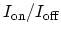ratio increases. At the same time, by increasing the gate-drain spacer length the parasitic capacitance between these two contacts decreases, which results in a reduced gate-delay time. Fig. 5.14 shows the effect of the gate-drain spacer length on the gate-delay time versus theratio, which indicates a significant performance improvement by increasing the gate-drain spacer length.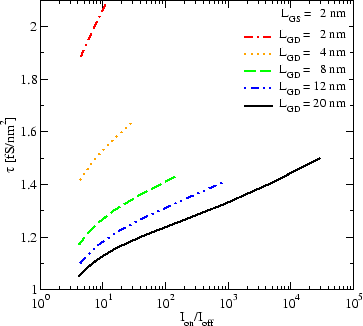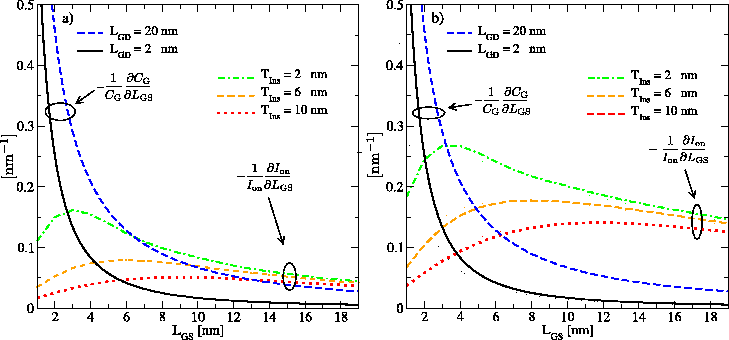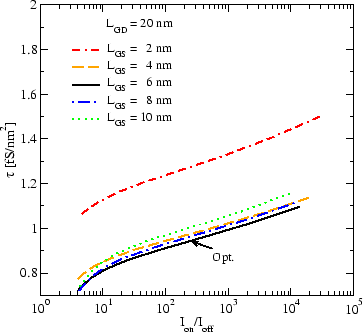Since the gate-delay time is proportional to the parasitic capacitance and inversely proportional to the on-current (5.1), there is an optimal value for the gate-source spacer length,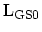, which minimizes the gate-delay time. The optimal value for the gate-source spacer length is achieved if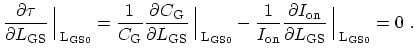(5.6)

Fig. 5.15 shows the sensitivity of the on-current with respect to the gate-source spacer length for devices with zero and positive barrier heights for electrons. For thinner insulators the width of the source-sided barrier decreases, resulting in a higher tunneling current contribution to the total current and a higher sensitivity of the on-current to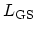(see Section 5.2.1).

The optimal gate-source spacer length for a device with zero barrier height for electrons is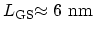for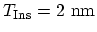and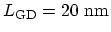. For devices with positive barrier heights the optimal value of the gate-source spacer length is smaller than that of a device with zero barrier height due to the higher sensitivity of the on-current with respect to the gate-source spacer length.

Note that the optimal value fordepends on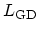. For small values ofthe gate-drain parasitic capacitance dominates the gate-source parasitic capacitance. Therefore, any further decrease of the gate-source spacer length does not improve the gate-delay time. As shown in Fig. 5.16, the optimal value of the gate-source spacer length for the given material and geometrical parameters results in optimized device characteristics.

M. Pourfath: Numerical Study of Quantum Transport in Carbon Nanotube-Based Transistors